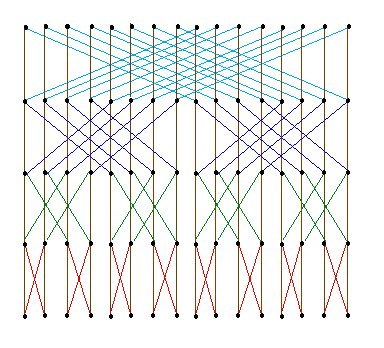# Purely Functional Data Structures & Algorithms : Fast Fourier Transform in QiIn this second post in this series we look at an implementation of the always useful Fast Fourier Transform.
(FFT) An algorithm for computing the Fourier transform of a set of discrete data values. Given a finite set of data points, for example a periodic sampling taken from a real-world signal, the FFT expresses the data in terms of its component frequencies. It also solves the essentially identical inverse problem of reconstructing a signal from the frequency data.
The FFT is a mainstay of numerical analysis. Gilbert Strang described it as “the most important algorithm of our generation”. The FFT also provides the asymptotically fastest known algorithm for multiplying two polynomials.
Our implementation comes in at just under 100 lines of code

``` Math
(declare atan [number --> number])
(define atan X -> (ATAN X))
(declare cos [number --> number])
(define cos X -> (COS X))
(declare sin [number --> number])
(define sin X -> (SIN X))
(tc +)
Complex numbers
(datatype complex
Real : number; Imag : number;
=============================
[Real Imag] : complex;)
(define complex-mult
{complex --> complex --> complex}
[R1 I1] [R2 I2] -> [(- (* R1 R2) (* I1 I2))
(+ (* R1 I2) (* I1 R2))])
{complex --> complex --> complex}
[R1 I1] [R2 I2] -> [(+ R1 R2) (+ I1 I2)])
(define complex-diff
{complex --> complex --> complex}
[R1 I1] [R2 I2] -> [(- R1 R2) (- I1 I2)])
Fast Fourier Transform
(define butterfly-list
{((list complex) * ((list complex) * (list complex)))
--> ((list complex) * ((list complex) * (list complex)))}
(@p X (@p X1 X2)) -> (if (empty? X)
(@p X (@p (reverse X1) (reverse X2)))
(butterfly-list
(@p (tail (tail X))
(define calc-results
{(((list complex) * (list (list complex))) *
((list complex) * (list complex)))
--> (((list complex) * (list (list complex))) *
((list complex) * (list complex)))}
(@p (@p [W WN] [YA YB]) (@p Y1 Y2)) ->
(if (and (empty? Y1) (empty? Y2))
(@p (@p [W WN] [(reverse YA) (reverse YB)]) (@p Y1 Y2))
(calc-results
(@p (@p [(complex-mult W WN) WN]
(@p (tail Y1) (tail Y2))))))
(define fft
{number --> complex --> (list complex) --> (list complex)
--> (list complex)}
1 WN X Y -> [(head X)]
N WN X Y -> (let M   (round (/ N 2))
Inp (butterfly-list (@p X (@p [] [])))
X1  (fst (snd Inp))
X2  (snd (snd Inp))
Y1  (fft M (complex-mult WN WN) X1 [])
Y2  (fft M (complex-mult WN WN) X2 [])
W   [1 0]
Res (calc-results (@p (@p [W WN] [[] []]) (@p Y1 Y2)))
(define dotimes-fft
{number --> number --> complex --> (list complex) --> (list complex)
--> (list complex)}
Iterations Size W Input Res ->
(if ( number --> (list complex)
--> (list complex)}
Iterations Size Input -> (let Pi    (* 4 (atan 1))
Theta (* 2 (/ Pi Size))
W     [(cos Theta) (* -1 (sin Theta))]
(dotimes-fft Iterations Size W Input [])))```

Let’s give it a spin …

``` Square wave test
(26-) (time (run-fft 100000 16
[[0 0] [1 0] [0 0] [1 0] [0 0] [1 0] [0 0] [1 0]
[0 0] [1 0] [0 0] [1 0] [0 0] [1 0] [0 0] [1 0]]))
Evaluation took:
2.999 seconds of real time
2.942718 seconds of total run time (2.798716 user, 0.144002 system)
[ Run times consist of 0.371 seconds GC time, and 2.572 seconds non-GC time. ]
98.13% CPU
6,282,874,678 processor cycles
1,641,619,888 bytes consed
[[8 0] [0.0 0.0] [0.0 0.0] [0.0 0.0]
[0.0 0.0] [0.0 0.0] [0.0 0.0] [0.0 0.0]
[-8 0] [0.0 0.0] [0.0 0.0] [0.0 0.0]
[0.0 0.0] [0.0 0.0] [0.0 0.0] [0.0 0.0]] : (list complex)```

All Qi code in this post is here.